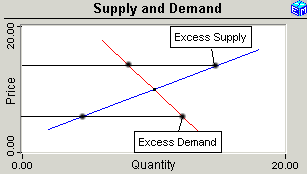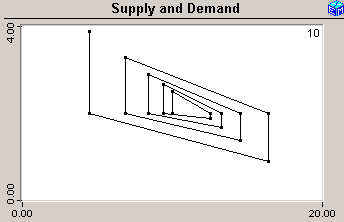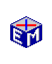The Cobweb Model

In the basic model of supply and demand, the price adjusts so that the quantity supplied and the quantity demanded are equal.  The precise mechanism that achieves this equilibrium is not always explicit.The Cobweb Model shows how achieving a supply and demand equilibrium might be so automatic if, as seems reasonable, the suppliers set the price and the consumers react with a quantity demanded.  For some slopes of the demand and supply curves, the equilibrium can be unstable.

The Cobweb Model is the classic demonstration that dynamic behavior by economic agents might not converge to a stable equilibrium with supply equal to demand.  This application provides two ways to graph the outcome and lets you experiment with the key parameter that determines whether the outcome is stable or not.

Movie:  The Cobweb Model (1 min, 34 seconds)The Cobweb Model
Model Link (The Getting Started page
explains how to activate the model links.)
Exercises (printable pdf)

The Inventory-Based Pricing Model relaxes the assumption that supply must equal demand to consider how maintaining an inventory might moderate the possible instability. Retail stores, for example, very often buy an inventory, set a price, and then wait to see what demand might be.

The Inventory-Based Pricing Model illustrates that the Cobweb Model might or might not be a realistic challenge to supply and demand equilibrium.  Introducing an inventory buffering the difference between supply and demand and letting prices respond to the level of the inventory can be sufficient to eliminate the instability observed for the basic Cobweb Model.The Cobweb Model
Model Link (The Getting Started page
explains how to activate the model links.)
Exercises (printable pdf)

Classic Economic Models

Microeconomics

Introduction
Overview of Micro Models

Macroeconomics

Introduction
Overview of Macro Models

Resources